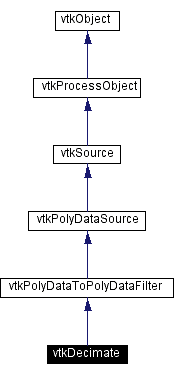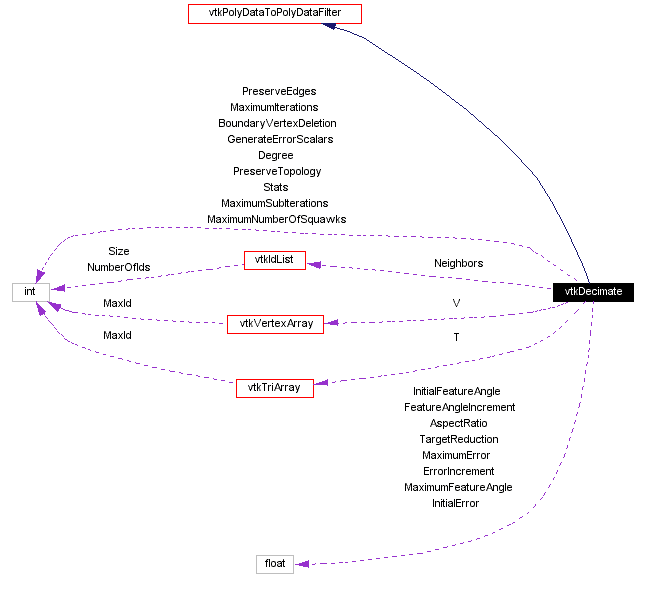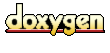Main Page   Class Hierarchy   Alphabetical List   Compound List   File List   Compound Members   File Members   Related Pages

# vtkDecimate Class Reference

reduce the number of triangles in a mesh. More...

`#include <vtkDecimate.h>`

Inheritance diagram for vtkDecimate:[legend]
Collaboration diagram for vtkDecimate:[legend]
List of all members.

## Public Methods

virtual const char * GetClassName ()
virtual int IsA (const char *type)
void PrintSelf (ostream &os, vtkIndent indent)
virtual void SetInitialError (float)
virtual float GetInitialError ()
virtual void SetErrorIncrement (float)
virtual float GetErrorIncrement ()
virtual void SetMaximumError (float)
virtual float GetMaximumError ()
virtual void SetTargetReduction (float)
virtual float GetTargetReduction ()
virtual void SetMaximumIterations (int)
virtual int GetMaximumIterations ()
virtual void SetMaximumSubIterations (int)
virtual int GetMaximumSubIterations ()
virtual void SetInitialFeatureAngle (float)
virtual float GetInitialFeatureAngle ()
virtual void SetFeatureAngleIncrement (float)
virtual float GetFeatureAngleIncrement ()
virtual void SetMaximumFeatureAngle (float)
virtual float GetMaximumFeatureAngle ()
virtual void SetGenerateErrorScalars (int)
virtual int GetGenerateErrorScalars ()
virtual void GenerateErrorScalarsOn ()
virtual void GenerateErrorScalarsOff ()
virtual void SetPreserveEdges (int)
virtual int GetPreserveEdges ()
virtual void PreserveEdgesOn ()
virtual void PreserveEdgesOff ()
virtual void SetBoundaryVertexDeletion (int)
virtual int GetBoundaryVertexDeletion ()
virtual void BoundaryVertexDeletionOn ()
virtual void BoundaryVertexDeletionOff ()
virtual void SetAspectRatio (float)
virtual float GetAspectRatio ()
virtual void SetPreserveTopology (int)
virtual int GetPreserveTopology ()
virtual void PreserveTopologyOn ()
virtual void PreserveTopologyOff ()
virtual void SetDegree (int)
virtual int GetDegree ()
virtual void SetMaximumNumberOfSquawks (int)
virtual int GetMaximumNumberOfSquawks ()

## Static Public Methods

vtkDecimate * New ()
int IsTypeOf (const char *type)
vtkDecimate * SafeDownCast (vtkObject *o)

## Protected Methods

vtkDecimate ()
~vtkDecimate ()
vtkDecimate (const vtkDecimate &)
void operator= (const vtkDecimate &)
void Execute ()
void CreateOutput (int numPts, int numTris, int numEliminated, vtkPointData *pd, vtkPoints *inPts)
int BuildLoop (int ptId, unsigned short int nTris, int *tris)
void EvaluateLoop (int &vtype, int &numFEdges, vtkLocalVertexPtr fedges[])
int CanSplitLoop (vtkLocalVertexPtr fedges, int numVerts, vtkLocalVertexPtr verts[], int &n1, vtkLocalVertexPtr l1[], int &n2, vtkLocalVertexPtr l2[], float &ar)
void SplitLoop (vtkLocalVertexPtr fedges, int numVerts, vtkLocalVertexPtr *verts, int &n1, vtkLocalVertexPtr *l1, int &n2, vtkLocalVertexPtr *l2)
void Triangulate (int numVerts, vtkLocalVertexPtr verts[])
int CheckError ()

## Protected Attributes

float InitialFeatureAngle
float FeatureAngleIncrement
float MaximumFeatureAngle
int PreserveEdges
int BoundaryVertexDeletion
float InitialError
float ErrorIncrement
float MaximumError
float TargetReduction
int MaximumIterations
int MaximumSubIterations
float AspectRatio
int Degree
int Stats [VTK_NUMBER_STATISTICS]
int GenerateErrorScalars
int MaximumNumberOfSquawks
int PreserveTopology
vtkIdListNeighbors
vtkVertexArrayV
vtkTriArrayT

## Detailed Description

reduce the number of triangles in a mesh.

Date:
2000/12/10 20:09:15
Revision:
1.47

vtkDecimate is a filter to reduce the number of triangles in a triangle mesh, while preserving the original topology and a forming good approximation to the original geometry. The input to vtkDecimate is a vtkPolyData object, and only triangles are treated. If you desire to decimate polygonal meshes, first triangulate the polygons with the vtkTriangleFilter object.

The algorithm proceeds as follows. Each vertex in the triangle list is evaluated for local planarity (i.e., the triangles using the vertex are gathered and compared to an "average" plane). If the region is locally planar, that is if the target vertex is within a certain distance of the average plane (i.e., the error), and there are no edges radiating from the vertex that have a dihedral angle greater than a user-specified edge angle (i.e., feature angle), and topology is not altered, then that vertex is deleted. The resulting hole is then patched by re-triangulation. The process continues over the entire vertex list (this constitutes an iteration). Iterations proceed until a target reduction is reached or a maximum iteration count is exceeded.

There are a number of additional parameters you can set to control the decimation algorithm. The Error ivar may be increased over each iteration with the ErrorIncrement. (These two variables have the largest effect.) Edge preservation (i.e., PreserveEdges ivar) may be disabled or enabled. You can turn on/off edge vertex deletion (i.e., BoundaryVertexDeletion ivar). (Edge vertices are vertices that lie along boundaries of meshes.) Sub iterations are iterations that are performed without changing the decimation criterion. The AspectRatio ivar controls the shape of the triangles that are created, and is the ratio of maximum edge length to minimum edge length. The Degree is the number of triangles using a single vertex. Vertices of high degree are considered "complex" and are never deleted.

Warning:
This implementation has been adapted for a global error bound decimation criterion. That is, the error is a global bound on distance to original surface. This is an improvement over the original Siggraph paper ("Decimation of Triangle Meshes", Proc Siggraph `92.)
Warning:
The algorithm has been extended with a special flag to allow topology modification. When the PreserveTopology flag is on, then the algorithm will preserve the topology of the original mesh. If off, the algorithm may close holes and/or collapse tunnels (i.e., form non-manifold attachments).
Examples:
vtkDecimate (examples)

Definition at line 179 of file vtkDecimate.h.

## Constructor & Destructor Documentation

 vtkDecimate::vtkDecimate ( ) ` [protected]`

 vtkDecimate::~vtkDecimate ( ) ` [protected]`

 vtkDecimate::vtkDecimate ( const vtkDecimate & ) ` [inline, protected]`
 Definition at line 271 of file vtkDecimate.h.

## Member Function Documentation

 vtkDecimate* vtkDecimate::New ( ) ` [static]`
 Instantiate object with no start, end, or progress methods. Reimplemented from vtkPolyDataSource.

 virtual const char* vtkDecimate::GetClassName ( ) ` [virtual]`
 Return the class name as a string. This method is defined in all subclasses of vtkObject with the vtkTypeMacro found in vtkSetGet.h. Reimplemented from vtkPolyDataToPolyDataFilter.

 int vtkDecimate::IsTypeOf ( const char * type ) ` [static]`
 Return 1 if this class type is the same type of (or a subclass of) the named class. Returns 0 otherwise. This method works in combination with vtkTypeMacro found in vtkSetGet.h. Reimplemented from vtkPolyDataToPolyDataFilter.

 virtual int vtkDecimate::IsA ( const char * type ) ` [virtual]`
 Return 1 if this class is the same type of (or a subclass of) the named class. Returns 0 otherwise. This method works in combination with vtkTypeMacro found in vtkSetGet.h. Reimplemented from vtkPolyDataToPolyDataFilter.

 vtkDecimate* vtkDecimate::SafeDownCast ( vtkObject * o ) ` [static]`
 Will cast the supplied object to vtkObject* is this is a safe operation (i.e., a safe downcast); otherwise NULL is returned. This method is defined in all subclasses of vtkObject with the vtkTypeMacro found in vtkSetGet.h. Reimplemented from vtkPolyDataToPolyDataFilter.

 void vtkDecimate::PrintSelf ( ostream & os, vtkIndent indent ) ` [virtual]`
 Methods invoked by print to print information about the object including superclasses. Typically not called by the user (use Print() instead) but used in the hierarchical print process to combine the output of several classes. Reimplemented from vtkSource.

 virtual void vtkDecimate::SetInitialError ( float ) ` [virtual]`
 Set the decimation error bounds. Expressed as a fraction of the longest side of the input data's bounding box.

 virtual float vtkDecimate::GetInitialError ( ) ` [virtual]`

 virtual void vtkDecimate::SetErrorIncrement ( float ) ` [virtual]`
 Set the value of the increment by which to increase the decimation error after each iteration.

 virtual float vtkDecimate::GetErrorIncrement ( ) ` [virtual]`

 virtual void vtkDecimate::SetMaximumError ( float ) ` [virtual]`
 Set the largest decimation error that can be achieved by incrementing the error.

 virtual float vtkDecimate::GetMaximumError ( ) ` [virtual]`

 virtual void vtkDecimate::SetTargetReduction ( float ) ` [virtual]`
 Specify the desired reduction in the total number of polygons. Because of various constraints, this level of reduction may not be realizable.

 virtual float vtkDecimate::GetTargetReduction ( ) ` [virtual]`

 virtual void vtkDecimate::SetMaximumIterations ( int ) ` [virtual]`
 Specify the maximum number of iterations to attempt. If decimation target is reached first, this value will not be reached.

 virtual int vtkDecimate::GetMaximumIterations ( ) ` [virtual]`

 virtual void vtkDecimate::SetMaximumSubIterations ( int ) ` [virtual]`
 Specify the maximum sub-iterations to perform. If no triangles are deleted in a sub-iteration, the sub-iteration process is stopped.

 virtual int vtkDecimate::GetMaximumSubIterations ( ) ` [virtual]`

 virtual void vtkDecimate::SetInitialFeatureAngle ( float ) ` [virtual]`
 Specify the mesh feature angles.

 virtual float vtkDecimate::GetInitialFeatureAngle ( ) ` [virtual]`

 virtual void vtkDecimate::SetFeatureAngleIncrement ( float ) ` [virtual]`
 Set/Get the angle by which to increase feature angle over each iteration.

 virtual float vtkDecimate::GetFeatureAngleIncrement ( ) ` [virtual]`

 virtual void vtkDecimate::SetMaximumFeatureAngle ( float ) ` [virtual]`
 Set the largest permissible feature angle.

 virtual float vtkDecimate::GetMaximumFeatureAngle ( ) ` [virtual]`

 virtual void vtkDecimate::SetGenerateErrorScalars ( int ) ` [virtual]`
 Turn on/off the generation of error scalars.

 virtual int vtkDecimate::GetGenerateErrorScalars ( ) ` [virtual]`

 virtual void vtkDecimate::GenerateErrorScalarsOn ( ) ` [virtual]`

 virtual void vtkDecimate::GenerateErrorScalarsOff ( ) ` [virtual]`

 virtual void vtkDecimate::SetPreserveEdges ( int ) ` [virtual]`
 Turn on/off the preservation of feature edges.

 virtual int vtkDecimate::GetPreserveEdges ( ) ` [virtual]`

 virtual void vtkDecimate::PreserveEdgesOn ( ) ` [virtual]`

 virtual void vtkDecimate::PreserveEdgesOff ( ) ` [virtual]`

 virtual void vtkDecimate::SetBoundaryVertexDeletion ( int ) ` [virtual]`
 Turn on/off the deletion of vertices on the boundary of a mesh.

 virtual int vtkDecimate::GetBoundaryVertexDeletion ( ) ` [virtual]`

 virtual void vtkDecimate::BoundaryVertexDeletionOn ( ) ` [virtual]`

 virtual void vtkDecimate::BoundaryVertexDeletionOff ( ) ` [virtual]`

 virtual void vtkDecimate::SetAspectRatio ( float ) ` [virtual]`
 Specify the maximum allowable aspect ratio during triangulation.

 virtual float vtkDecimate::GetAspectRatio ( ) ` [virtual]`

 virtual void vtkDecimate::SetPreserveTopology ( int ) ` [virtual]`
 Turn on/off whether to preserve the topology of the original mesh. If off, hole elimination and non-manifold attachment can occur.

 virtual int vtkDecimate::GetPreserveTopology ( ) ` [virtual]`

 virtual void vtkDecimate::PreserveTopologyOn ( ) ` [virtual]`

 virtual void vtkDecimate::PreserveTopologyOff ( ) ` [virtual]`

 virtual void vtkDecimate::SetDegree ( int ) ` [virtual]`
 If the number of triangles connected to a vertex exceeds "Degree", then the vertex is considered complex and is never deleted. (NOTE: the complexity of the triangulation algorithm is proportional to Degree^2.)

 virtual int vtkDecimate::GetDegree ( ) ` [virtual]`

 virtual void vtkDecimate::SetMaximumNumberOfSquawks ( int ) ` [virtual]`
 Control the printout of warnings. This flag limits the number of warnings regarding non-manifold geometry and complex vertices. If set to zero, no warnings will appear.

 virtual int vtkDecimate::GetMaximumNumberOfSquawks ( ) ` [virtual]`

 void vtkDecimate::operator= ( const vtkDecimate & ) ` [inline, protected]`
 Definition at line 272 of file vtkDecimate.h.

 void vtkDecimate::Execute ( ) ` [protected, virtual]`
 Reimplemented from vtkSource.

 void vtkDecimate::CreateOutput ( int numPts, int numTris, int numEliminated, vtkPointData * pd, vtkPoints * inPts ) ` [protected]`

 int vtkDecimate::BuildLoop ( int ptId, unsigned short int nTris, int * tris ) ` [protected]`

 void vtkDecimate::EvaluateLoop ( int & vtype, int & numFEdges, vtkLocalVertexPtr fedges[] ) ` [protected]`

 int vtkDecimate::CanSplitLoop ( vtkLocalVertexPtr fedges, int numVerts, vtkLocalVertexPtr verts[], int & n1, vtkLocalVertexPtr l1[], int & n2, vtkLocalVertexPtr l2[], float & ar ) ` [protected]`

 void vtkDecimate::SplitLoop ( vtkLocalVertexPtr fedges, int numVerts, vtkLocalVertexPtr * verts, int & n1, vtkLocalVertexPtr * l1, int & n2, vtkLocalVertexPtr * l2 ) ` [protected]`

 void vtkDecimate::Triangulate ( int numVerts, vtkLocalVertexPtr verts[] ) ` [protected]`

 int vtkDecimate::CheckError ( ) ` [protected]`

## Member Data Documentation

 float vtkDecimate::InitialFeatureAngle` [protected]`
 Definition at line 276 of file vtkDecimate.h.

 float vtkDecimate::FeatureAngleIncrement` [protected]`
 Definition at line 277 of file vtkDecimate.h.

 float vtkDecimate::MaximumFeatureAngle` [protected]`
 Definition at line 278 of file vtkDecimate.h.

 int vtkDecimate::PreserveEdges` [protected]`
 Definition at line 279 of file vtkDecimate.h.

 int vtkDecimate::BoundaryVertexDeletion` [protected]`
 Definition at line 280 of file vtkDecimate.h.

 float vtkDecimate::InitialError` [protected]`
 Definition at line 281 of file vtkDecimate.h.

 float vtkDecimate::ErrorIncrement` [protected]`
 Definition at line 282 of file vtkDecimate.h.

 float vtkDecimate::MaximumError` [protected]`
 Definition at line 283 of file vtkDecimate.h.

 float vtkDecimate::TargetReduction` [protected]`
 Definition at line 284 of file vtkDecimate.h.

 int vtkDecimate::MaximumIterations` [protected]`
 Definition at line 285 of file vtkDecimate.h.

 int vtkDecimate::MaximumSubIterations` [protected]`
 Definition at line 286 of file vtkDecimate.h.

 float vtkDecimate::AspectRatio` [protected]`
 Definition at line 287 of file vtkDecimate.h.

 int vtkDecimate::Degree` [protected]`
 Definition at line 288 of file vtkDecimate.h.

 int vtkDecimate::Stats[VTK_NUMBER_STATISTICS]` [protected]`
 Definition at line 289 of file vtkDecimate.h.

 int vtkDecimate::GenerateErrorScalars` [protected]`
 Definition at line 290 of file vtkDecimate.h.

 int vtkDecimate::MaximumNumberOfSquawks` [protected]`
 Definition at line 291 of file vtkDecimate.h.

 int vtkDecimate::PreserveTopology` [protected]`
 Definition at line 292 of file vtkDecimate.h.

 vtkIdList* vtkDecimate::Neighbors` [protected]`
 Definition at line 293 of file vtkDecimate.h.

 vtkVertexArray* vtkDecimate::V` [protected]`
 Definition at line 294 of file vtkDecimate.h.

 vtkTriArray* vtkDecimate::T` [protected]`
 Definition at line 295 of file vtkDecimate.h.

The documentation for this class was generated from the following file:

Generated on Wed Nov 21 12:47:27 2001 for VTK by1.2.11.1 written by Dimitri van Heesch, © 1997-2001